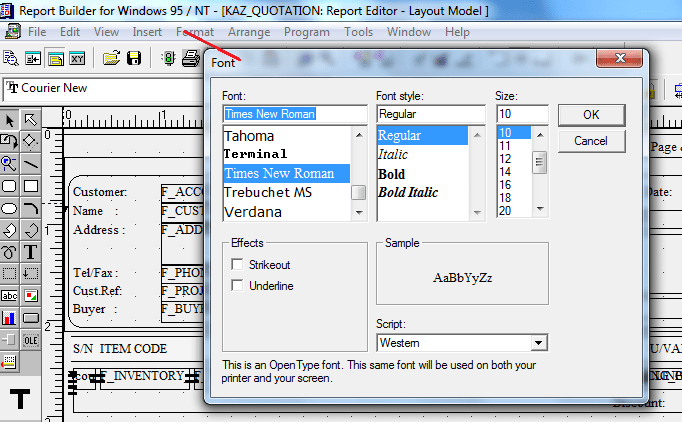# Oracle Application R12, 7 Buckets Supplier Aging SQL

```[sourcecode language='sql'  padlinenumbers='true']
Select supplier_number, vendor_name,
sum(amount_remaining) amount_remaining,
sum(b0) "<=30",
sum(b1) "31-60",
sum(b2) "61-90",
sum(b3) "91-180",
sum(b4) "181-270",
sum(b5) "271-360",
sum(b6) ">360"
FROM(
SELECT i.invoice_date,
round(nvl(ps.amount_remaining,0)*nvl(i.exchange_rate, 1),3) amount_remaining,
i.vendor_id, supp.vendor_name, supp.segment1 supplier_number,
CASE
when trunc(trunc(sysdate))-i.invoice_date <= 30 then
NVL(round(nvl(ps.amount_remaining,0)*nvl(i.exchange_rate, 1),3),0)
END b0
,
CASE
when trunc(sysdate)-i.invoice_date BETWEEN 31 AND 60 then
NVL(round(nvl(ps.amount_remaining,0)*nvl(i.exchange_rate, 1),3),0)
END b1,
CASE
when trunc(sysdate)-i.invoice_date BETWEEN 61 AND 90 then
NVL(round(nvl(ps.amount_remaining,0)*nvl(i.exchange_rate, 1),3),0)
END b2,
CASE
when trunc(sysdate)-i.invoice_date BETWEEN 91 AND 180 then
NVL(round(nvl(ps.amount_remaining,0)*nvl(i.exchange_rate, 1),3),0)
END b3  ,
CASE
when trunc(sysdate)-i.invoice_date BETWEEN 181 AND 270 then
NVL(round(nvl(ps.amount_remaining,0)*nvl(i.exchange_rate, 1),3),0)
END b4
,
CASE
when trunc(sysdate)-i.invoice_date BETWEEN 271 AND 360 then
NVL(round(nvl(ps.amount_remaining,0)*nvl(i.exchange_rate, 1),3),0)
END b5     ,
CASE
when trunc(sysdate)-i.invoice_date > 360 then
NVL(round(nvl(ps.amount_remaining,0)*nvl(i.exchange_rate, 1),3),0)
END b6
FROM ap_payment_schedules ps, ap_invoices i, ap_suppliers supp
WHERE     i.invoice_id = ps.invoice_id
AND ps.org_id = :P_ORG_ID -- Security takes care of this part, only for other reporting reqs
AND i.vendor_id = supp.vendor_id
AND i.cancelled_date IS NULL
AND ps.amount_remaining <> 0
)
GROUP BY   supplier_number, vendor_name
ORDER BY  vendor_name
[/sourcecode]
```

And as usual, the main view is a protected repository, hence you have to initialize the security part in order to fetch data

```[sourcecode language='sql' ]
begin
MO_GLOBAL.SET_POLICY_CONTEXT('S',:P_ORG_ID);
end ;

begin
--fnd_global.apps_initialize(:P_USER_ID,:P_RESP_ID,:P_RESP_APPL_ID);
fnd_global.apps_initialize(1353, 50854, 200);
end;

begin
MO_GLOBAL.INIT('SQLAP'); --Payables
--MO_GLOBAL.INIT('PO');
end;
[/sourcecode]
```

Now enjoy another quality stuff from us :)

PS for Kuwait requirements, we have rounded the figures to 3 digits, alter the script to suite your reporting requirements.

regards,

# Oralce apps reports, instance producing gibberish character mapping

We are @ the final stage of implementing Oracle e-business suit 12 and recently came across a peculiar font mapping problem with custom developed reports. We use both Developer 6i reports developer as well as 10g reports developer.
Once the reports are tested and uploaded to the apps instance, the outputs were always producing gibrish (PDF outputs especially). However after few days of experimenting, finally we found a work around (hopefully)!

Following are the tips: